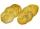# Integer equation + greatest common divisor (GCD) - examples

1. Diofant 2Is equation ? solvable on the set of integers Z?
2. SeedcakeSeedcake costs 44 cents. How many minimum seedcakes we must buy that we can pay in cash only whole euros?
3. Diofant equationIn the set of integers (Z) solve the equation: ? Write result with integer parameter ? (parameter t = ...-2,-1,0,1,2,3... if equation has infinitely many solutions)

We apologize, but in this category are not a lot of examples.
Do you have an interesting mathematical example that you can't solve it? Enter it, and we can try to solve it.

To this e-mail address, we will reply solution; solved examples are also published here. Please enter e-mail correctly and check whether you don't have a full mailbox.

Do you want to calculate greatest common divisor two or more numbers? Do you solve Diofant problems and looking for a calculator of Diofant integer equations?